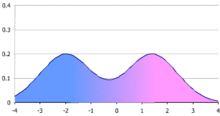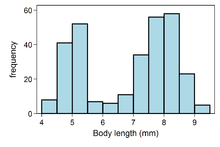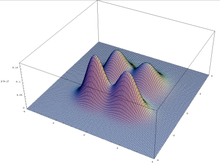# Bimodal distribution

Bimodal distributionFigure 1. A simple bimodal distribution, in this case a mixture of two normal distributions with the same variance but different means. The figure shows the probability density function (p.d.f.), which is an average of the bell-shaped p.d.f.s of the two normal distributions.Figure 2. Histogram of body lengths of 300 weaver ant workers.Figure 3. A bivariate, multimodal distribution.

In statistics, a bimodal distribution is a continuous probability distribution with two different modes. These appear as distinct peaks (local maxima) in the probability density function, as shown in Figure 1.

Examples of variables with bimodal distributions include the time between eruptions of certain geysers, the color of galaxies, the size of worker weaver ants, the age of incidence of Hodgkin's lymphoma, the speed of inactivation of the drug isoniazid in US adults, and the absolute magnitude of novae.

## Mixture distributions

A bimodal distribution most commonly arises as a mixture of two different unimodal distributions (i.e. distributions having only one mode). In other words, the bimodally distributed random variable X is defined as Y with probability α or Z with probability (1 − α), where Y and Z are unimodal random variables and 0 < α < 1 is a mixture coefficient. For example, the bimodal distribution of sizes of weaver ant workers shown in Figure 2 arises due to existence of two distinct classes of workers, namely major workers and minor workers. In this case, Y would be the size of a random major worker, Z the size of a random minor worker, and α the proportion of worker weaver ants that are major workers.

A mixture of two unimodal distributions with differing means is not necessarily bimodal, however. The combined distribution of heights of men and women is sometimes used as an example of a bimodal distribution, but in fact the difference in mean heights of men and women is too small relative to their standard deviations to produce bimodality. A mixture of two normal distributions with equal standard deviations is bimodal only if their means differ by at least twice the common standard deviation.

## Summary statistics

Bimodal distributions are a commonly-used example of how summary statistics such as the mean, median, and standard deviation can be deceptive when used on an arbitrary distribution. For example, in the distribution in Figure 1, the mean and median would be about zero, even though zero is not a typical value. The standard deviation is also larger than deviation of each normal distribution.

## Multimodality

More generally, a multimodal distribution is a continuous probability distribution with two or more modes, as illustrated in Figure 3.

Wikimedia Foundation. 2010.

### Look at other dictionaries:

• bimodal distribution — See central tendency (measures of) …   Dictionary of sociology

• bimodal — imodal adj. (Statistics) having or occurring with two {modes}; having two maxima; of a curve or distribution. {unimodal} [WordNet 1.5] …   The Collaborative International Dictionary of English

• bimodal — See length frequency distribution …   Fisheries — dictionary

• bimodal — bimodality, n. /buy mohd l/, adj. 1. having or providing two modes, methods, systems, etc. 2. Statistics. (of a distribution) having or occurring with two modes. Cf. unimodal. 3. Transp. pertaining to or suitable for transportation involving the… …   Universalium

• bimodal — adjective having or involving two modes, in particular (of a statistical distribution) having two maxima …   English new terms dictionary

• bimodal — bi•mod•al [[t]baɪˈmoʊd l[/t]] adj. 1) cvb having or providing two modes, methods, systems, etc 2) sta (of a distribution in statistics) having or occurring with two modes • Etymology: 1900–05 bi mo•dal′i•ty, n …   From formal English to slang

• bimodal — adjective of a distribution; having or occurring with two modes • Ant: ↑unimodal • Topics: ↑statistics …   Useful english dictionary

• Occupancy frequency distribution — In macroecology and community ecology, an occupancy frequency distribution (OFD) is the distribution of the numbers of species occupying different numbers of areas. It was first reported in 1918 by the Danish botanist Christen C. Raunkiær in… …   Wikipedia

• Binomial distribution — Probability distribution name =Binomial type =mass pdf cdf Colors match the image above parameters =n geq 0 number of trials (integer) 0leq p leq 1 success probability (real) support =k in {0,dots,n}! pdf ={nchoose k} p^k (1 p)^{n k} ! cdf =I {1… …   Wikipedia

• U-quadratic distribution — Probability distribution name =U Quadratic type =density pdf cdf parameters =a: a in ( infty,infty)b: b in (a, infty) or alpha: alphain (0,infty)eta: eta in ( infty,infty), support =xin [a , b] ! pdf =alpha left ( x eta ight )^2 cdf ={alpha… …   Wikipedia